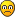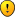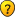## [SOLVED] Problem with anam.fit during Gauss. anam. calc.

If you have problems using RGeostats package or if you simply need some help on an unlisted subject.

### [SOLVED] Problem with anam.fit during Gauss. anam. calc.

Dear FriendsI have a problem during Gaussian anamorphosis calculating before make Turning Bands simulation (with using simtub).
I prepared next steps as below:
Code: Select all
`library(RGeostats)name=file.choose()B4=read.csv(name,header=T,na="MISS")class(B4)val=db.create(B4)val=db.locate(val,4:6,"x")val=db.locate(val,7,"z")model.anam <- anam.fit(val,type="gaus",nbpoly=100,draw=T)`

and obtained following error:

No Anam Slot available: all entries are used (1)
All Entries are released for future use

The function is interrupted
ERROR: Error in R_anam_fit_hermitian

I spent many hours on it and made almost everything like defined new object in class anam etc. I can't use anam.z2y and anam.y2z if I don't have anam.fit so I am stuckCould you help me solved this problem? Many thanks for attentionAK-Ch

Posts: 3
Joined: Fri Jul 11, 2014 2:08 pm
Location: Wrocław, Poland

### Re: Problem with anam.fit during Gaussian anamorphosis calcu

The error that you mentionned is a problem that will be solved in future version.
In fact, it comes from a horrible interface (between R and C) problem. Each R-object is attached to C using a slot. There is a limited number of slots available (10 for 'db', ... , 1 for 'anam').
This slot is opened at the beginning of a procedure (such as anam.fit) to transmit all the information contained in the R-object to the corresponding C-structure. At the end of the procedure, this slot is freed and released.
This is true UNLESS the procedure fails: then the slot is not released.
When the pile of slots is full, there is no more slot available (of a given type) and the next procedure will fail (with the message that you gave).

The workaround is:
- getting out and in R will solve this ... but it is painful
- the piles can be released manually using the command: constant.reset()

Cheers
Didier Renard

Posts: 294
Joined: Thu Sep 20, 2012 4:22 pm

### Re: Problem with anam.fit during Gaussian anamorphosis calcu

Hello Didier,

Many thanks for your replayNow is everything working on, when I did it manually, by using your suggested commandI have last question in this topic. Namely, what settings are given as default (about Anamorphosis) in simtub functionWhen I set NA in parameter anam and flag.back will be equal FALSE, what could be done with raw data during simulation? Then I also obtain results with different scale (more symmetric/gaussian). If I set anam=model.anam and flag.back=TRUE, I have to also use anam.y2z function (it seems works the same like flag.back=TRUE) after calculated simtub? Below I pasted my code to make my questions clear.
Thank you a lot for your time and given answers.

Best regards,
Ania

Code: Select all
`library(RGeostats)name=file.choose()B4=read.csv(name,header=T,na="MISS")class(B4)val=db.create(B4)val=db.locate(val,4:6,"x")val=db.locate(val,7,"z")model.anam <- anam.fit(val,type="gaus",nbpoly=100,draw=T)val <- anam.z2y(val,anam=model.anam)grid3D=db.create(flag.grid=TRUE,nx=c(48,55,6),x0=c(20,10,3),dx=c(1,1,3),ndim=3,nvar=1,autoname=TRUE)grid3D=migrate(val,grid3D)vario3D_4dir=vario.calc(val,lag=5,nlag=15,dir=c(0,45,90,135))plot(vario3D_4dir,npairdw=T,npairpt=T)x11()m4dir=model.auto(vario3D_4dir,struct=melem.name(c(1,2,3)))plot(m4dir,add=T,vario=vario3D_4dir)x11()moving.neigh3D=neigh.create(ndim=3,type=2,flag.sector=T,flag.aniso=T,flag.rotation=F,nmini=1,nmaxi=3,radius=c(120,120,100))result3D=simtub(val,grid3D,model=m4dir,neigh=moving.neigh3D,anam=model.anam,flag.back=TRUE,seed=232131,nbtuba=100,nbsimu=100)result3D <- anam.y2z(result3D,anam=model.anam)plot(result3D,col=topo.colors(100),pos.legend=5,xlab="x [m]",ylab="y [m]")`
AK-Ch

Posts: 3
Joined: Fri Jul 11, 2014 2:08 pm
Location: Wrocław, Poland

### Re: Problem with anam.fit during Gaussian anamorphosis calcu

Hello

To answer to your question, we have to start by the correct point.
When using simulations (using Turning Bands method for example), we NEED to turn the data in the gaussian framework (this is the only valid framework where simtub can run). Actually, it is not tested (difficult stuff) and left to the responsability of the user.
As a complementary idea, if you use simulations (where the conditioning is performed using Ordinary Kriging), we can even accept data which would be SHIFTED gaussian (mean different from 0).

The result of simtub are simulation outcomes, essentially in gaussian space. Then, in order to ease the task of the user, we included the flag.back possibility (which requires the definition of anam): it simply calls anam.y2z internally.

Hope this will help
Didier Renard

Posts: 294
Joined: Thu Sep 20, 2012 4:22 pm

### Re: Problem with anam.fit during Gaussian anamorphosis calcu

Hello Didier,

Thanks a lot for quick responseNow, it is all clear. Generally, I know geostatistical simulation methods in theory, but I did not sure how it is implemented here with which assumptions. It was important to me to know how correctly prepare input data and parameters. Yours answer dispelled all my doubtsMerci beacoupBest regards,
Ania
AK-Ch

Posts: 3
Joined: Fri Jul 11, 2014 2:08 pm
Location: Wrocław, Poland